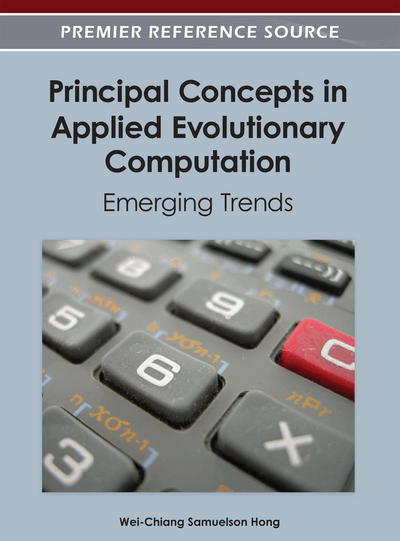# A Heuristic Approach for Multi Objective Distribution Feeder Reconfiguration: Using Fuzzy Sets in Normalization of Objective Functions

Armin Ebrahimi Milani (Islamic Azad University, Iran) and Mahmood Reza Haghifam (Tarbiat Modarres University, Iran)
DOI: 10.4018/978-1-4666-1749-0.ch007
Available
\$37.50
No Current Special Offers

## Abstract

The reconfiguration is an operation process used for optimization with specific objectives by means of changing the status of switches in a distribution network. This paper presents an algorithm for network recon-figuration based on the heuristic rules and fuzzy multi objective approach where each objective is normalized with inspiration from fuzzy set to cause optimization more flexible and formulized as a unique multi objective function. Also, the genetic algorithm is used for solving the suggested model, in which there is no risk of non-linear objective functions and constraints. The effectiveness of the proposed method is demonstrated through several examples in this paper.
Chapter Preview
Top

## 1. Introduction

In the original configuration of a distribution network there are some normally open (N.O.) and some normally closed (N.C) switches. Clearly, in order to reach specific purposes, we can close some of N.O switches and open corresponding N.C ones.

The whole mentioned operation for reaching a new optimized configuration is named “Reconfiguration of distribution networks”.

In general, reconfiguration methods can be divided to two groups: “General” and “Specific”. In the specific methods, one initial answer is obtained and is used in a specific algorithm to reach further answers up to an improvement point considering the constraints of the problem. In general methods, an algorithm is used for solving the problem and a large range of answer are obtained, among which by performing an operation the most improved answer is selected as a final one.

So far, several different techniques have been presented to resolve the network reconfiguration problem. For instance, Civanlar et al. in (Civanlar, Grainger, Yin, & Lee, 1998) described a formula to estimate the loss change resulting from the transfer of a group of loads from one feeder to another feeder. In Baran and Wu (1989) a heuristic algorithm was employed to minimize the power loss and load balancing, while Liu, Lee, and Vu (1989) developed a global optimality condition for the problem and two solution algorithms.

Shirmohammadi et al. (Shirmohammadi & Hong, 1989) described a technique for the reconfiguration of distribution networks to decrease their resistive line losses and included results pertaining to large scale system examples. In Wang, Chiang, and Darling (1996) explicit loss reduction and line flow formulas were developed to determine efficiently the switching operations.

Recently, AI-based approaches have been described to solve the problem and the results of such methods as the expert system (Liu, Lee, & Venkata, 1988), Genetic algorithm (Nara, Shiose, Kitagawa, & Ishihara, 1992), neural network (Kim, Ko, & Jung, 1993), simulated annealing (Chang & Kuo, 1994), evolutionary programming (EP) (Lin, Cheng, & Tsay, 1999), and fuzzy logic (Zhou, Shirmohammadi, & Liu, 1997; Prasad, Ranjan, Sahoo, & Chaturvedi, 2005) are all encouraging. Where their methodologies in (Zhou, Shirmohammadi, & Liu, 1997) combined the optimization techniques with heuristic rules and fuzzy logic for efficiency and robust performance and (Prasad, Ranjan, Sahoo, & Chaturvedi, 2005) presented a fuzzy mutated genetic algorithm for optimal reconfiguration of radial distribution systems. This method involves a new chromosome representation of the network and a fuzzy mutation control for an effective search of solution space.

As it is clear, in the most introduced methods a uni-objective function is considered for solving the problem and due to the importance of the power loss minimization, reconfiguration with the purpose of reduction in loss is highly signified.

In this essay four objectives, included in one multi-objective function with weight factor related to each objective are considered as following:

• 1.

Minimization of the system’s power loss;

• 2.

Minimization of the deviation of nodes voltage;

• 3.

Minimization of the branch current constraint violation;

• 4.